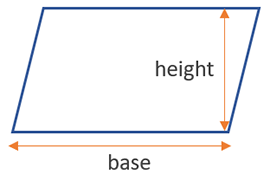# Area of a Parallelogram Calculator

Use this calculator to easily calculate the area of a parallelogram by its base and height.

Share calculator:

Embed this tool:
get code

## Area of a parallelogram formula

The formula for the area of a parallelogram is base x height. Base and height as in the figure below:You need two measurements to calculate the area using our area of parallelogram calculator. The formula is actually the same as that for a rectangle, since the area of a parallelogram is basically the area of a rectangle that has as its sides the parallelogram's base and height. This can be easily understood visually - if you use the height to cut a triangle from the shape, then spin it 180° and move it to the other side, it will perfectly match, and the shape will be that of a rectangle.

As with other similar calculations, it is important to multiply in identical units. The result is always the square unit: for example, square feet, square inches, square yards or square miles. In the metric systems you can get square centimeters, square meters, square kilometers, and others.

## Area of a Parallelogram calculation

You need to take two measurements - the base is easily measured, while for the height you would need to build a right angle at one of the parallelogram's angles. It doesn't matter which one, as long as one of the angle's arms is the base.

Then you can apply the simple multiplication formula above, making sure both lengths are of the same unit.

## Example: find the area of a parallelogram

To find the area of a parallelogram-shaped surface requires information about its base and height. It does not matter which side you take as base, as long as the height you use is perpendicular to it. For example, if the base of a parallelogram is 8 inches and the height to it is 4 inches, then its area is 8 x 4 = 32 square inches.

#### Cite this calculator & page

If you'd like to cite this online calculator resource and information as provided on the page, you can use the following citation:
Georgiev G.Z., "Area of a Parallelogram Calculator", [online] Available at: https://www.gigacalculator.com/calculators/area-of-parallelogram-calculator.php URL [Accessed Date: 01 Apr, 2023].